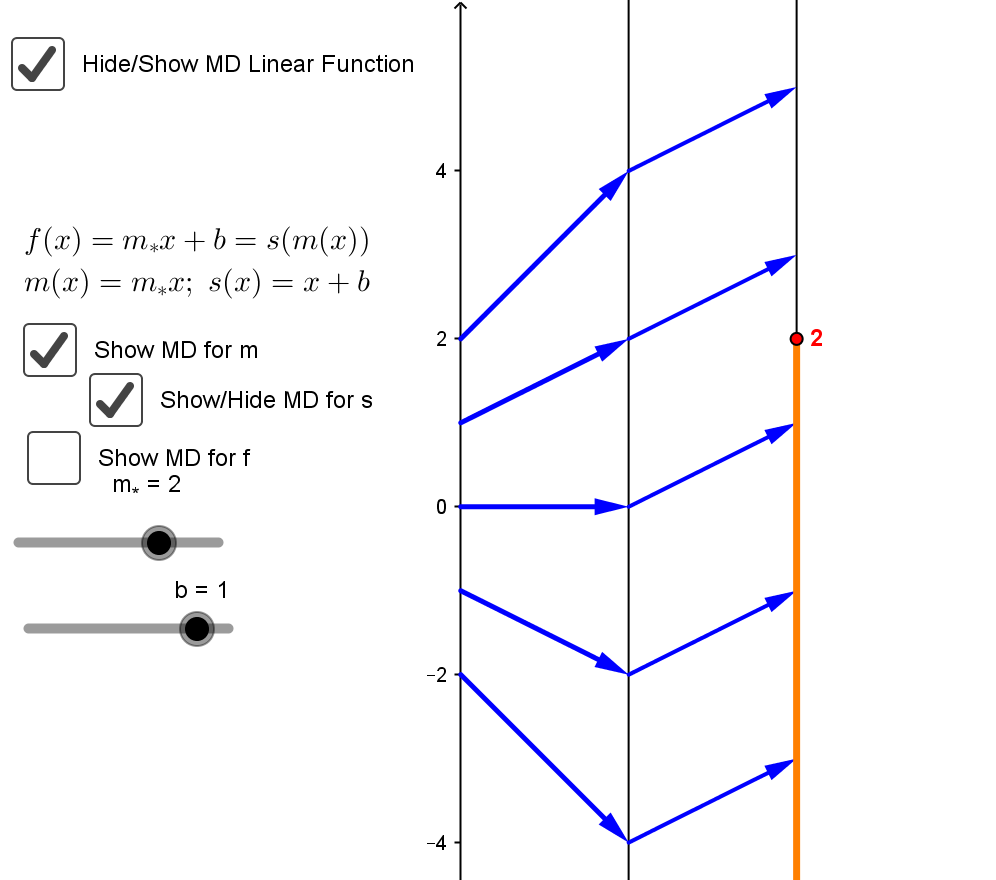Example LInEQ.2.  Suppose $2x + 1\le 2$. Solve the inequality for $x$.
Solution: The inequality can be solved using the common algebraic steps:
$2x + 1 \le 2$
$\ \ \ \ -1 = -1$

$2x\ \ \ \ \ \le 1$
$\frac 12 (2x) \le \frac 12 (1)$

$\ \ \ \ \ x \le \frac 12$

This conforms with the symbolic result,  $x \le \frac {C- B} A$  where $A=2, B = 1$, and $C = 2$. So the solution is $x \le \frac {2- 1} 2 = \frac {1} 2.$

Comment: We can consider the expression on the left hand side of the equation as a linear function of $x$ giving $f(x) = 2x + 1 = s(m(x))$ where $m(x) =2x$ and $s(x)=x+1$
The problem can be restated now: To find a value for $x$ where $f(x) \le 2$.
This problem and its solution can be visualized with mapping diagrams for the linear functions $f$ treated as a composition of $m$ followed by $s$.On the GeoGebra mapping diagram for the composition, find $2$ on the final target axis.

Undo $s$ by subtracting $1$ from $2$. This corresponds to going back on the diagram from $2$ on the final axis to $2-1=1$ on the middle axis, reversing the arrow that would go from $1$ to $2$ visualizing $s$.
Thus if $m(x) = 1$ then $f(x) = s(m(x))=s(1)=2$

Undo $m$ by multiplying $1$ by $\frac 12$. This corresponds to going back on the diagram from $1$ on the middle axis to $\frac 12$ on the source axis, reversing the arrow that would go from $\frac 12$ to $1$ visualizing $m$.
Thus when $x\le\frac 12$, $f(x) \le s(m(\frac 12))=s(1)=2$

On the GeoGebra sketch you can change  the values of $m=A$ and $b=B$ (with the sliders) and $C$ (currently $2$ on the target axis) to see other linear inequalities $Ax + B \le C$ are solved visually with the mapping diagram.